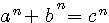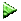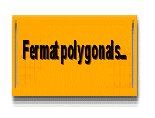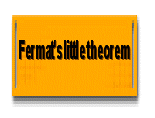#### Vers la version françaiseSite dedicated to Fermat's arithmetic -

## You'll find on this site a lot of livened illustrations on the magic of numbers

On Diophante and Fermat's tracks...

For the lastest version of shemath.com please go on here and translate with the included tool

# The FERMAT guess

Since the Antiquity, mathematicians try to resolve enigmae on the key of figures (prime, square numbers of numbers, Pi).

After having studied and used, as computer specialist, their diverse works during several years, I was attracted by Fermat's Theorem ( 1601-1665 ) and especially by the small intriguing sentence which he annotated in the margin of his copy of "Arithmetica" of Diophante: " I found a wonderful demonstration of this proposition, but the margin is too narrow to contain it ".

This Fermat's annotation hint to. Fermat wrote that if this equation has an infinite number of solutions when n is = 2, it has no solution when the power is superior to 2 .

This theorem will be demonstrate by Andrew Wiles from Princeton's university,with Richard Taylor's help , and  published in 1995 (Annals of Mathematics .)

All writings or internet said that the Pierre de Fermat'annotate is either false, or unknown this day, because the Andrew Wiles's demonstration uses mathematicals tools which Mr. de Fermat could not credibly have considering the knowledge of his time.

My goal is to show on this site that Pierre de Fermat had well and truly found , I'm using simple tools and just a little of imagination.

# Primary Goals:Through these studies, supply educational tools (Animations, graphs) to popularize and visualize Arithmetic.Prove that Pierre de Fermat have all the necessary knowledge to announce his guess, (by-products, modular arithmetic,primary numbers, differential arithmetic, geometry, Elongated numbers and new drawing) and that the solution was so evident for him as he did not write it.Put new guesses discovered thanks to the study of the Arithmetic of Fermat on prime numbersProve that odd / even numbers have not the same behavior.

# 1/ Infinity of A² + B² = C² : Topologic squares guess

By the  Fermat's sentence " but the margin is too narrow to contain it " I have imagined this simple graphic demonstration enlarging the margin of Fermat.

First ,consider that c²=b²+a² is equal to b²= c² - a² (or a² = c² - b²): By dividing a square into even or odd 'strips' I create 2 new squares:

## EVEN Topology

By  'b' square , 2 new squares have been created , a smallest square  'a'  showed in center and a biggers square 'c'. sample: if b=4 , a=3 , c= 5

## ODD Topology

By ' a ' square, 2 new biggest squares have been created  ' b'  and 'c'.

Sample under: a=5 , b=12 , c= 13

You can do this experience with a simple square of paper cut in 4 and gathered  as above!!! What demonstrates very simply the Pythagoras' theorem (C ² = In ² + B ²)

Mathematicly , by this way , you can produce an infinity of squares using 2 formulas (odd and even) with one parameter

## SectionForm all the pythagoriennes suites with only 2 formulaes (include 29-21-20) and 1 primary parameterFounding parity: it's 2 formulaes , even and odd, that produce different parities (in fact 2 different topologyRight-angled primary triangles: thoses triangles can be enlarged whith a constant parameter 'k'

#From this new principle and papers of Fermat, this study tries to demonstrate that Fermat had can be found this logical solution by a cleverly way.Topologic volumes.

# Polygonals numbersAnimated démonstrations - Bases studies - GlossaryFormulaes...Binary !!!

# Fermat's small theoremNew guess based on small Fermat's theorem.Modular studies

# Tools and computer's software Schemath.com - Windjax tmAnimated mathematics (for beginners)Equations developments software (Free download for P.C )"Fermat's calculator": Use square topologic formulae producing a lot of right angled triangles and prove the new "Fermat's logical solution" for pow 3 and 4Matrices

By Patrick Stoltz :

le 19/02/2009  dépôt INPI n°: 343319 (topologics squares)- schemath.com[Accueil Schemath.com][index schemath.com]